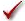## What does "hit constraint" mean?

Prism reports "Hit constraint" when the best-fit value of one or more parameters is right at the limit of a constraint.

When a fit hits a constraint, the results are unlikely to provide useful information. If you had a solid reason to constrain a parameter within a range of values, it ought to end up in that range. If the fit hit the constraint limit, that means the true best-fit value is some value forbidden by the constraint.

Prism does not compute confidence and prediction bands when a parameter hit a constraint. The best-fit values are not a local minimum, so any attempt to compute confidence or prediction bands would give misleading results.  Prism does compute the confidence intervals for the other parameters (the ones that didn't hit a constraint) but these need to be viewed with caution.

When a fit ends up hitting a constraint, it is likely that you set the constraint incorrectly. So the first thing to do is make sure the constraint is sensible and correctly entered. For example, if you mistakenly set a constraint that a rate constant K must be less than or equal to zero, Prism's nonlinear regression might converge with the best-fit value equalling zero and report that you hit the constraint. Fix the constrain to be greater than or equal to zero, and the fit will work fine.

Another possibility is to change the constraint from an inequality (Bottom>0) to a constant constraint (Bottom=0). You'll get the same parameter values, but difference confidence intervals, and you can get confidence and prediction bands.

ChecklistDid you enter the constraint correctly? Did you mix up "<" and ">".Did you enter the model correctly?Are you asking Prism to do the impossible -- too many parameters for your data?If your model has two phases or components, consider switching to a one phase or component model.